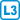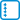## Edge omission in cubic graph

Let $$G$$ be a cubic graph without bridges. Prove it for each its edge there is a perfect matching that avoids it.

• #### Hint

Remove the edge from the graph and check Tutte’s condition, e.g. by a contradiction.

Note that at least 3 edges lead from the odd components.

• #### Solution

Let $$G$$ be a cubic graph without bridges. Cubic graphs satisfy Tutte’s condition, that is, for every $$S \subseteq V (G)$$ holds that $$| S | \ge C_o (G, S)$$, where $$C_o (G, S)$$ stands for the number of odd components of the graph $$G-S$$.

When we remove the edge $$e$$, we assume for a contradiction that $$G-e$$ does not have a perfect matching and therefore Tutte’s condition does not apply to it and there is a set $$S \subseteq V (G)$$ such that $$| S | < C_o (G-e, S)$$

If $$e$$ had both ends in $$S$$ or both ends in the same component, then $$C_o (G, S) = C_o (Ge, S)$$ and we get the contradiction immediately. So necessarily $$e$$ connects two odd components of $$G-e$$. They become one even by returning $$e$$ in $$G$$. Thus $$C_o (G-e, S) = C_o (G, S) +2$$.

These two components are not only connected to each other by the egde $$e$$, but are also connected to the set $$S$$. Because in the graph $$G-e$$ these are odd components, an odd number of edges should lead into them according to the parity principle. Since the $$G$$ graph has no bridges, there are at least three edges connecting each odd component (in both $$G$$ and $$G-e$$). Similarly, at least two edges lead to even components. Thus, at least four edges connect the merged component in $$G$$. In total of at least $$3 C_o (G, S) +4$$ edges lead to $$S$$.

Conversely, in $$G-e$$, it holds that $$| S | < C_o (G-e, S)$$. Both sides are integers, so $$3 | S | < 3 C_o (G-e, S) -2$$.

Because $$G$$ is cubic, vertices of $$S$$ are incident with at most $$3 | S |$$ edges. Therefore, for the number of edges $$\#e$$ incident with $$S$$ in the graph $$G$$ the following inequality holds: $3 C_o (G, S) +4 \le \#e \le 3 | S | <3 C_o (G-e, S) -2 = 3 (C_o (G, S) +2) -2 = 3 C_o (G, S) +4,$ which is the desired contradiction.